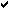# * Astronomy

 Home -> Astronomy News -> YouTube -> Newton's laws of motion
Post Info TOPIC: Newton's laws of motionLPosts: 131433
Date:
 RE: Newton's laws of motion Permalink Newton's laws of motion are three physical laws that form the basis for classical mechanics. They describe the relationship between the forces acting on a body and its motion due to those forces. They have been expressed in several different ways over nearly three centuries, and can be summarised as follows:First law: The velocity of a body remains constant unless the body is acted upon by an external force.Second law: The acceleration a of a body is parallel and directly proportional to the net force F and inversely proportional to the mass m, i.e., F = ma.Third law: The mutual forces of action and reaction between two bodies are equal, opposite and collinear. __________________LPosts: 131433
Date:
 Permalink Look Around You: Newtons Laws of Motion  __________________
 Page 1 of 1  sorted by Oldest FirstNewest First